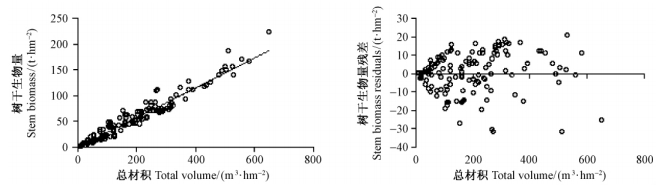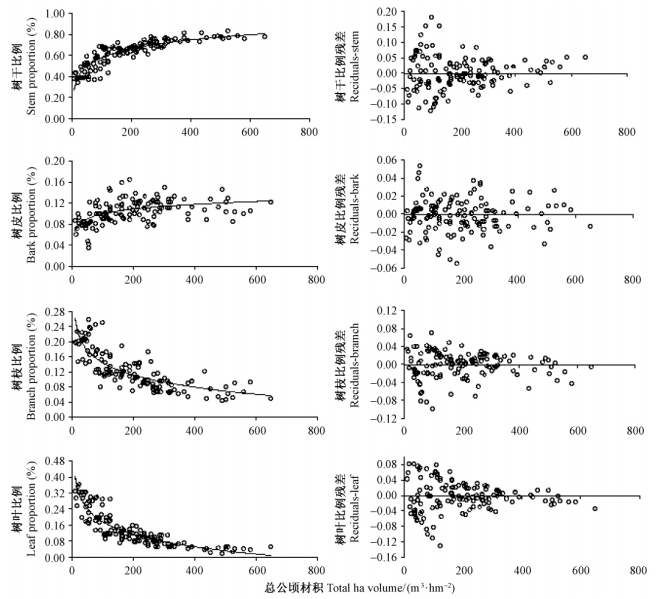﻿ 八种亚热带森林类型乔木层地上生物量分配模型
 林业科学2014, Vol. 50Issue (9): 1-9PDF
DOI: 10.11707/j.1001-7488.20140901
0

#### 文章信息

Fu Tian, Zhu Jianhua, Xiao Wenfa, Zeng Lixiong

Above-Ground Biomass Distribution Models for Arbor Layer of Eight Subtropical Forest Types

Scientia Silvae Sinicae, 2014, 50(9): 1-9.
DOI: 10.11707/j.1001-7488.20140901

### 作者相关文章

Above-Ground Biomass Distribution Models for Arbor Layer of Eight Subtropical Forest Types
Fu Tian, Zhu Jianhua, Xiao Wenfa, Zeng Lixiong
Research Institute of Forest Ecosystem Environment and Protection, CAF Beijing 100091
Abstract: In this study, the volume-to-biomass conversion models and the proportion models for biomass components of 8 subtropical forest types in China were established by using the power function model and multinomial logit model, respectively. The results show that the fitting results of the volume-to-biomass conversion models for every forest type except the evergreen broad-leaved forest exhibit significant relations (R2>0.5, P<0.001) between volume and biomass, and the predicting residuals increase with the increment of the independent variable value. The root mean square errors are all controlled within 6.520~23.123 t·hm-2. The testing accuracy is between 31.14%-91.79%, which are all above 70% except for evergreen broad-leaved forest. On the other hand, the fitting results of proportion models for the biomass components reach significant level (P <0.05) except for the evergreen broad-leaved forest, and the predicting residuals decrease with the increased independent variable value. The root mean square errors of stem, bark, branch and leaf biomass proportion of total tree biomass are all less than 0.1, that is 0.031~0.085, 0.005~0.041, 0.029~0.103 and 0.016~0.083. The prediction accuracy reaches 91.04%~96.14%, 62.71%~94.48%, 63.70%~94.47%, and －8.86%~83.92%, respectively. The volume-to-biomass conversion models and the proportion models for biomass components in this paper are reasonable models for the 8 subtropical forest types except for the evergreen broad-leaved forest, and the obtained model parameters also can provide a reference for studying biomass distribution of the other subtropical forests
Key words: power function    multinomial logit model    volume-to-biomass conversion    biomass proportion

1 材料来源2 模型方法 2.1 样地材积与树干生物量的关系模型

 $W = na{\left({{{\bar D}^2}\bar H} \right)^b}$ (1)
 $V = na'{\left({{{\bar D}^2}\bar H} \right)^{b'}}$ (2)

 $\begin{array}{l} W = na{\left[ {\frac{1}{{na'}}na'{{\left({{{\bar D}^2}\bar H} \right)}^{b'}}} \right]^{b/b'}} = \\ \frac{n}{{{{\left(n \right)}^{b/b'}}}} \cdot \frac{a}{{{{\left({a'} \right)}^{b/b'}}}}{\left[ {na'{{\left({{{\bar D}^2}\bar H} \right)}^{b'}}} \right]^{b/b'}} \end{array}$ (3)

 $W = {n^{1 - d}}c{V^d}$ (4)

 ${W_s} = {a_s} \times {V^{{b_s}}}$ (5)

2.2 林木器官生物量比例模型

 $\begin{array}{l} {P_s} = 1/\left[ {{e^{{a_1} + {a_2} \times V + {a_3} \times \ln \left({V + 5} \right)}}} \right. + \\ {e^{{b_1} + {b_2} \times V + {b_3} \times \ln \left({V + 5} \right)}} + \\ e\left. {^{{c_1} + {c_2} \times V + {c_3} \times \ln \left({V + 5} \right)}} \right] \end{array}$ (6)
 $\begin{array}{l} {P_b} = \left[ {{e^{{a_1} + {a_2} \times V + {a_3} \times \ln \left({V + 5} \right)}}} \right./\\ {e^{{a_1} + {a_2} \times V + {a_3} \times \ln \left({V + 5} \right)}} + \\ {e^{{b_1} + {b_2} \times V + {b_3} \times \ln \left({V + 5} \right)}} + \\ e\left. {^{{c_1} + {c_2} \times V + {c_3} \times \ln \left({V + 5} \right)}} \right]; \end{array}$ (7)
 $\begin{array}{l} {P_{br}} = \left[ {{e^{{b_1} + {b_2} \times V + {b_3} \times \ln \left({V + 5} \right)}}} \right./\\ {e^{{a_1} + {a_2} \times V + {a_3} \times \ln \left({V + 5} \right)}} + \\ {e^{{b_1} + {b_2} \times V + {b_3} \times \ln \left({V + 5} \right)}} + \\ e\left. {^{{c_1} + {c_2} \times V + {c_3} \times \ln \left({V + 5} \right)}} \right]; \end{array}$ (8)
 $\begin{array}{l} {P_f} = \left[ {{e^{{c_1} + {c_2} \times V + {c_3} \times \ln \left({V + 5} \right)}}} \right./\\ {\left[ e \right.^{{a_1} + {a_2} \times V + {a_3} \times \ln \left({V + 5} \right)}} + \\ {e^{{b_1} + {b_2} \times V + {b_3} \times \ln \left({V + 5} \right)}} + \\ e\left. {^{{c_1} + {c_2} \times V + {c_3} \times \ln \left({V + 5} \right)}} \right]. \end{array}$ (9)

2.3 模型评价与精度检验

 ${\rm{RMSE = }}\sqrt {\frac{{\sum {{{\left({y - {y_c}} \right)}^2}} }}{n}}$ (10)

 $P = 1 - {\rm{MAPE = }}\left({1 - \frac{1}{m}\sum {\frac{{\left| {y - {y_c}} \right|}}{y}} } \right)\times 100\%$ (11)

3 结果与分析 3.1 材积—生物量转换图 1 材积-生物量转换模型拟合效果(杉木林) Fig. 1 Results of volume-to-biomass conversion model(Chinese fir forest)
3.2 林木器官生物量比例图 2 林木器官生物量比例模型拟合效果(杉木林) Fig. 2 Results of proportion models for biomass components(Chinese fir forest)3.3 精度检验4 结论与讨论

  程瑞梅,肖文发,李建文,等. 1999.三峡库区森林植被分类系统初探. 环境与开发, 14 (2): 4-8.(1)  方精云,陈平安,赵淑清,等. 2002.中国森林生物量的估算: 对Fang等Science一文(Science, 2001,291:2320-2322)的若干说明.植物生态学报,26(2): 243-249.(1)  冯仲科,罗旭,石丽萍. 2005. 森林生物量研究的若干问题及完善途径.世界林业研究, 18(3): 25-28.(1)  巨文珍,王新杰,孙玉军. 2011. 长白落叶松林龄序列上的生物量及碳储量分配规律. 生态学报,31(4): 1139-1148.(1)  李海奎,雷渊才,曾伟生. 2011. 基于森林清查资料的中国森林植被碳储量. 林业科学,47(7): 7-12.(1)  骆期邦,曾伟生,贺东北,等. 1999. 立木地上部分生物量模型的建立及其应用研究.自然资源学报, 14(3): 271-277.(1)  饶雄,张景雄,丁翼星. 2008. 基于多项logit模型的土地覆被分层分类方法研究. 武汉大学学报: 信息科学版, 33(11): 1166-1169.(1)  唐守正,张会儒,胥辉. 2000. 相容性生物量模型的建立及其估计方法研究. 林业科学,36(Sp.1): 19-27.(1)  王仲锋. 2006. 森林生物量建模与精度分析.北京: 北京林业大学博士学位论文.(1)  胥辉. 1999. 一种与材积相容的生物量模型. 北京林业大学学报,21(5):32-36.(1)  肖文发,李建文,于长青,等. 2000.长江三峡库区陆生动植物生态. 重庆: 西南师范大学出版社, 5-11.(1)  曾立雄,王鹏程,肖文发,等. 2008.三峡库区主要植被生物量与生产力分配特征. 林业科学, 44(8): 16-22.(1)  Brown S, Lugo A E. 1984. Biomass of tropical forests: a new estimate based on forest volumes. Science, 223(4642): 1290-1293.(1)  Dixon R K, Brown S L, Houghton R A, et al. 1994. Carbon pools and flux of global forest ecosystems. Science, 263(5144): 185-190.(1)  Fang J, Chen A, Peng C, et al. 2001. Changes in forest biomass carbon storage in China between 1949 and 1998. Science, 292(5525): 2320-2322.(1)  Whittaker R H, Likens G E. 1975. Methods of assessing terrestrial productivity. New York: Springer-Verlag, 305-328.(1)